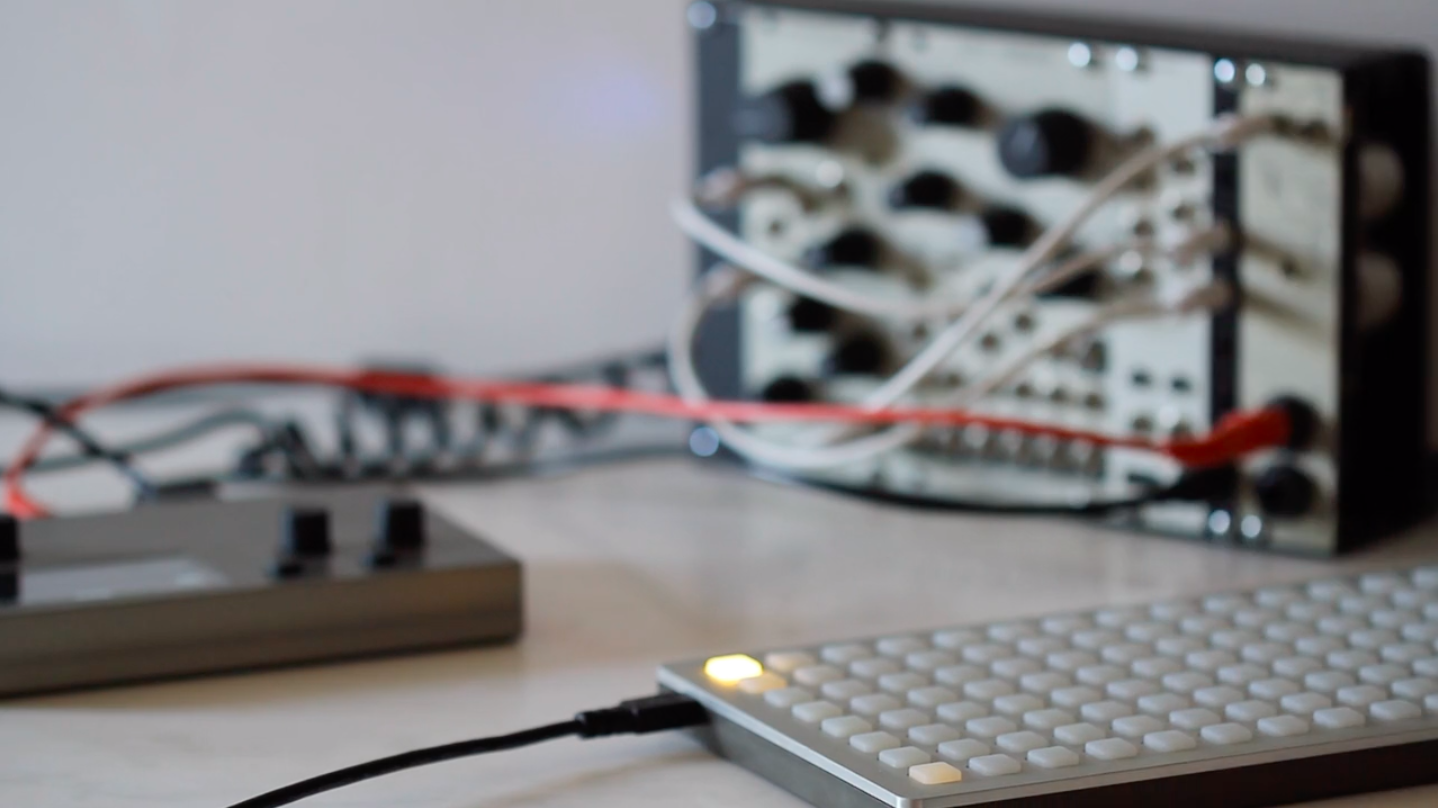# Rising: crow Studies

crow serves as a CV and ii interface for norns.

Before venturing further, it may be helpful to first explore the norns studies to provide context for how to integrate crow’s functionality.

crow will automatically be detected and interfaced upon connection to norns.
Presently, only a single crow is supported.

sections

## 1. Output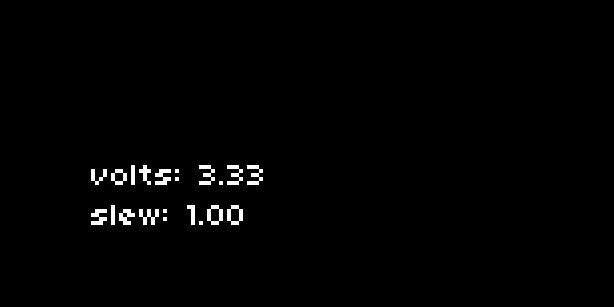Run `1-output.lua`. Connect crow output 1 to an oscillator pitch or similar.

This sets up a knob and screen interface for two very simple commands:

``````crow.output.volts = 3.33
crow.output.slew = 0.1
``````

This sets output 1 to 3.33v, slewing over 0.1 seconds.

Crow’s voltage range is -5.0 to 10.0 for outputs 1 to 4.

## 2. Input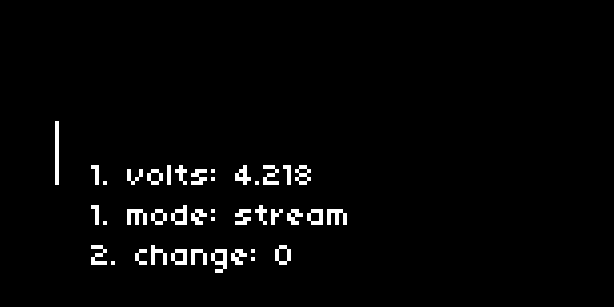Run `2-input.lua`.

• Connect an LFO output to crow input 1. K2 will capture the current value. K3 will toggle `stream` mode on and off.
• Connect the same cable to input 2 which is set up to trigger a `change` function on each transition.

Inputs have several modes:

• `stream`: the input is reported at a fixed interval.
• `change`: configurable low/high transitions are reported.
• `none`: inputs are read only with a manual query.

### Stream

First we set the function for incoming data, and then set the mode:

``````function process_stream(v)
print("input stream: "..v)
end

crow.input.stream = process_stream
crow.input.mode("stream", 0.1)
``````

`process_stream` will be called every 0.1 seconds, printing the value of crow input 1.

### Change

Again we create a function to handle the input change, and set the mode:

``````function process_change(v)
print("input change: "..v)
end

crow.input.change = process_change
crow.input.mode("change", 1.0, 0.1, "both")
``````

`process_change` will be called whenever input 1 crosses 1.0 volts with a hysteresis of 0.1.

If the input is rising, the value reported will be 1. If falling, it will be 0.

The last parameter when setting the mode can have three values: `"rising"`, `"falling"`, or “`both"`.

### Query

What if we want to manually query the input voltage?

We can manually query the input when `mode` is set to `"none"`.

``````function process_stream(v)
print("input stream: "..v)
end

crow.input.stream = process_stream
crow.input.mode("none")

crow.input.query()
``````

`process_stream` will be called each time `crow.input.query()` is called, returning the voltage at crow input 1.

## 3. ii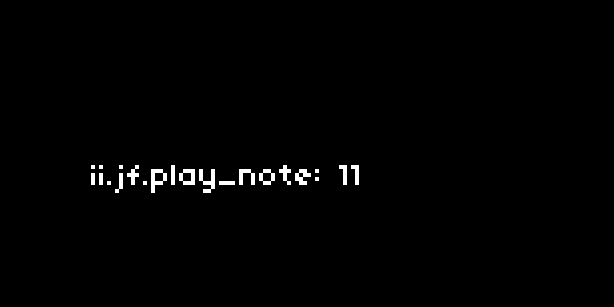Run `3-ii.lua`.

Attach a Just Friends via ii. Be sure to align the GND pins. K2 will play an ascending note, K3 plays a random note.

To change JF’s mode and play a note:

``````crow.ii.jf.mode(1)
crow.ii.jf.play_note(3)
``````

Crow can also query values from the ii bus. If you have an Ansible connected running Kria, you can query the current preset like this:

``````crow.ii.kria.event = function(i,v)
print("kria event:",i,v)
end

crow.ii.kria.get("preset")
``````

See the reference section for a full table of supported ii devices and commands.

## 4. shapes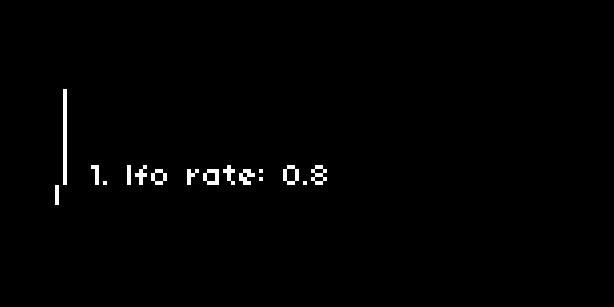Run `4-shapes.lua`. Crow output 1 is an LFO, output 2 is an envelope. K2 will randomize the LFO speed. K3 will trigger the envelope. Voltage output is displayed as meters on the left.

Crow can generate and loop multipoint envelopes:

``````-- start at 0, rise to 5V over 0.1 seconds, fall to 1V over 2 seconds
crow.output.action = "{ to(0,0), to(5,0.1), to(1,2) }"
``````

To start (and restart) this action:

``````crow.output()
``````

Shapes can be repeated:

``````crow.output.action = "times( 4, { to(0,0), to(5,0.1), to(1,2) } )"
``````

And also looped:

``````crow.output.action = "loop( { to(0,0), to(5,0.1), to(1,2) } )"
``````

Actions can be interrupted at any time by setting a fixed voltage, for example:

``````crow.output.volts = 0
``````

There are a few predefined shapes, such as LFO:

``````-- LFO rate of 1, amplitude of 5V
crow.output.action = "lfo(1,5)"
``````

### Query

It is possible to read the current value of an output using a query:

``````function out(v)
print("crow output: "..v)
end

crow.output.query()
``````

Each time `query` is called, crow will send a value to the function `receive`. The script uses this technique to create a realtime scope of the outputs on the norns screen.

## 5. construct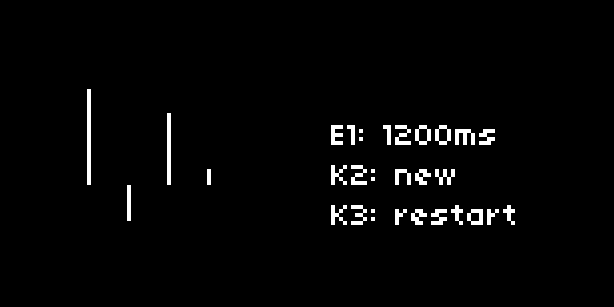Run `5-construct.lua`. Crow outputs 1-4 are 5-segment looping envelopes, each with its own unique shape and trajectory. E1 will change the timebase from which these LFOs are constructed. K2 will construct new LFOs at the specified timebase. K3 will restart the LFOs from 0V. The current Voltage output is displayed as meters on the left.

crow can speak a slope language, referred to as ASL. In the previous script, we learned how ASL `to` command can be used to create multipoint envelopes using Voltage and time. `to` also has a third argument for shape, which defines how we move from voltage to voltage across time. If we do not define the shape, `to` commands default to linear movement.

nb. This script uses some extended techniques, so don’t worry if it feels a little heady.

``````shapes = {'linear','sine','logarithmic','exponential','now','wait','over','under','rebound'}
``````

Using random, we query shapes from that table and generate Voltages and durations for each of segments. This constructs the `to` commands which will structure our evolving waves:

``````wave.to = to(0,0.4,under)
wave.to = to(-3.655,1.2,logarithmic)
wave.to = to(4.922,0.6,sine)
wave.to = to(2.536,1.2,linear)
wave.to = to(0,0.6,exponential)
``````

We concatenate these into a single statement:

``````wave.full = "loop( { "..wave.to..","..wave.to..","..wave.to..","..wave.to..","..wave.to.."} )"
``````

…which becomes:

``````wave.full = "loop( { to(0,0.4,under),to(-3.655,1.2,logarithmic),to(4.922,0.6,sine),toto(2.536,1.2,linear),to(0,0.6,exponential)} )"
``````

And finally, assign it to a crow output:

``````crow.output.action = wave.full
``````

## Reference

### Output

``````crow.output[x].volts = y         -- set output x (1 to 4) to y (-5.0 to 10.0) volts
crow.output[x].slew = y          -- set output x slew time to y

crow.output[x].action =
"{ to(volt,time,shape), ... , to(volt,time,shape) }"    -- series of segments
"times( x, { ... } )"                                   -- repeat segments x times
"loop( { ... } )"                                       -- loop segments indefinitely
"lfo(time,level,shape)"
"pulse(time,level,polarity)"
"ar(attack,release,shape)"

crow.output[x].query()           -- query current output x value
crow.output[x].receive           -- function called by query x
crow.output[x]()                 -- run the current action
``````

### Input

``````crow.input[x].stream             -- function called by "stream" mode and query
crow.input[x].change             -- function called by "change" mode

crow.input[x].mode("none")       -- set input x to query only
crow.input[x].mode("stream", rate)      -- set input x to stream mode at specified rate
crow.input[x].mode("change", thresh, hyst, edge) -- set input x to change mode
-- specify threshold, hysteresis, and edge ("rising", "falling", or "both")

crow.input[x].query()            -- queries current value of input x
``````

### ii

``````crow.ii.pullup(state)       -- enable/disable pullups (true/false)

-- crow
-- commands
crow.ii.crow.output( channel, level )
crow.ii.crow.slew( channel, time )
crow.ii.crow.call1( arg )
crow.ii.crow.call2( arg1, arg2 )
crow.ii.crow.call3( arg1, arg2, arg3 )
crow.ii.crow.call4( arg1, arg2, arg3, arg4 )

-- ansible
-- commands
crow.ii.ansible.trigger( channel, state )
crow.ii.ansible.trigger_toggle( channel )
crow.ii.ansible.trigger_pulse( channel )
crow.ii.ansible.trigger_time( channel, time )
crow.ii.ansible.trigger_polarity( channel, polarity )
crow.ii.ansible.cv( channel, volts )
crow.ii.ansible.cv_slew( channel, time )
crow.ii.ansible.cv_offset( channel, volts )
crow.ii.ansible.cv_set( channel, volts )

-- request params
crow.ii.ansible.get( 'trigger', channel )
crow.ii.ansible.get( 'trigger_time', channel )
crow.ii.ansible.get( 'trigger_polarity', channel )
crow.ii.ansible.get( 'cv', channel )
crow.ii.ansible.get( 'cv_slew', channel )
crow.ii.ansible.get( 'cv_offset', channel )

crow.ii.ansible.event = function( e, data )
if e == 'trigger' then
-- handle trigger param here
elseif e == 'trigger_time' then
elseif e == 'trigger_polarity' then
elseif e == 'cv' then
elseif e == 'cv_slew' then
elseif e == 'cv_offset' then
end
end

-- ansible kria
crow.ii.kria.preset( number )
crow.ii.kria.pattern( number )
crow.ii.kria.scale( number )
crow.ii.kria.period( time )
crow.ii.kria.position( track, param, pos )
crow.ii.kria.loop_start( track, param, pos )
crow.ii.kria.loop_length( track, param, pos )
crow.ii.kria.reset( track, param )
crow.ii.kria.mute( track, state )
crow.ii.kria.toggle_mute( track )
crow.ii.kria.clock( track )

-- request params
crow.ii.kria.get( 'preset' )
crow.ii.kria.get( 'pattern' )
crow.ii.kria.get( 'scale' )
crow.ii.kria.get( 'period' )
crow.ii.kria.get( 'position', track, param )
crow.ii.kria.get( 'loop_start', track, param )
crow.ii.kria.get( 'loop_length', track, param )
crow.ii.kria.get( 'reset', track )
crow.ii.kria.get( 'mute', track )
crow.ii.kria.get( 'cv', track )

crow.ii.kria.event = function( e, data )
if e == 'preset' then
-- handle preset param here
elseif e == 'pattern' then
elseif e == 'scale' then
elseif e == 'period' then
elseif e == 'position' then
elseif e == 'loop_start' then
elseif e == 'loop_length' then
elseif e == 'reset' then
elseif e == 'mute' then
end
end

-- commands

-- request params

crow.ii.meadowphysics.event = function( e, data )
if e == 'preset' then
-- handle preset param here
elseif e == 'stop' then
elseif e == 'scale' then
elseif e == 'period' then
elseif e == 'cv' then
end
end

-- jf
-- commands
crow.ii.jf.trigger( channel, state )
crow.ii.jf.run_mode( mode )
crow.ii.jf.run( volts )
crow.ii.jf.transpose( pitch )
crow.ii.jf.vtrigger( channel, level )
crow.ii.jf.mode( mode )
crow.ii.jf.tick( clock-or-bpm )
crow.ii.jf.play_voice( channel, pitch/divs, level/repeats )
crow.ii.jf.play_note( pitch/divs, level/repeats )
crow.ii.jf.god_mode( state )
crow.ii.jf.retune( channel, numerator, denominator )
crow.ii.jf.quantize( divisions )

-- w/
-- commands
crow.ii.wslash.record( active )
crow.ii.wslash.play( direction )
crow.ii.wslash.loop( state )
crow.ii.wslash.cue( destination )

-- request params
crow.ii.wslash.get( 'record' )
crow.ii.wslash.get( 'play' )
crow.ii.wslash.get( 'loop' )
crow.ii.wslash.get( 'cue' )